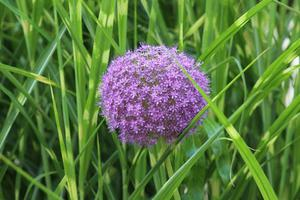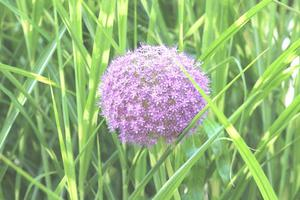Java Program to Increase or Decrease Brightness of an Image

• Last Updated : 06 Jan, 2022

Before understanding how the brightness is adjusted in any image first we have to understand how an image is represented. image is represented in the 2D form of a pixel, a pixel is the smallest element of an image. the value of pixel at any point corresponds to the intensity of light of the photons striking at that point. every pixel stores N-bit unsigned int value where N is the depth of the image (8-bit image, 16-bit image, 24-bit image). the value of pixel can vary from 0 to 255 for the 8-bit image where 0 corresponds to black and 255 corresponds to white. each pixel color is made up of a combination of RGB values. Brightness is nothing but how much the light perceive by an image.

Brightness of an image can be increase or decrease by just increasing or decreasing the RGB value of an image pixel.the value of brightness will usually be in the range of -255 to +255. Negative values will darken the image and positive values will brighten the image.

Steps:

We need to follow 3 steps sequentially as listed below as follows:

1. Read the image using ImageIO class
2. Getting the width and height of the image and loop through each and every pixel
3. Getting the RGB value of each pixel to add the amount of brightness

Implementation:

Consider a sample arbitrary input image with which we will be playing to increase or either away decrease its contrast to illustrate change.photo.jpg

Example

Java

 // Java Program to Increase or Decrease Brightness of an// Image import java.io.*; class GFG {     // Method 1    // Helper method to method 2    public static int Truncate(int value)    {         if (value < 0) {            value = 0;        }        else if (value > 255) {            value = 255;        }        return value;    }     // Method 2    // To adjust the brightness of image    public static void AdjustBrightness(String inpPath,                                        String outPath)        throws IOException    {         // Taking image path and reading pixels        File f = new File(inpPath);        BufferedImage image = ImageIO.read(f);         // Declaring an array for spectrum of colors        int rgb[];         // Setting custom brightness        int brightnessValue = 25;         // Outer loop for width of image        for (int i = 0; i < image.getWidth(); i++) {             // Inner loop for height of image            for (int j = 0; j < image.getHeight(); j++) {                 rgb = image.getRaster().getPixel(                    i, j, new int);                 // Using(calling) method 1                int red                    = Truncate(rgb + brightnessValue);                int green                    = Truncate(rgb + brightnessValue);                int blue                    = Truncate(rgb + brightnessValue);                 int arr[] = { red, green, blue };                 // Using setPixel() method                image.getRaster().setPixel(i, j, arr);            }        }         // Throwing changes over the image as read above        ImageIO.write(image, "jpg", new File(outPath));    }     // Method 3    // Main driver method    public static void main(String[] args) throws Exception    {         // Passing images present on directory on local        // machine        String inputPath = "E:\\photo.jpg";        String outputPath = "E:\\outphoto.jpg";         // Calling method 2 inside main() to        // adjust the brightness of image        AdjustBrightness(inputPath, outputPath);    }}

Output:  Bright Imageoutphoto.jpg

My Personal Notes arrow_drop_up